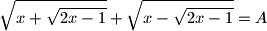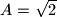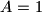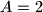### IMO Shortlist 1959 problem 2

Kvaliteta:
Avg: 2.0
Težina:
Avg: 3.0
For what real values of$x$ isgiven

a)$A=\sqrt{2}$;

b)$A=1$;

c)$A=2$,

where only non-negative real numbers are admitted for square roots?
Source: Međunarodna matematička olimpijada, shortlist 1959